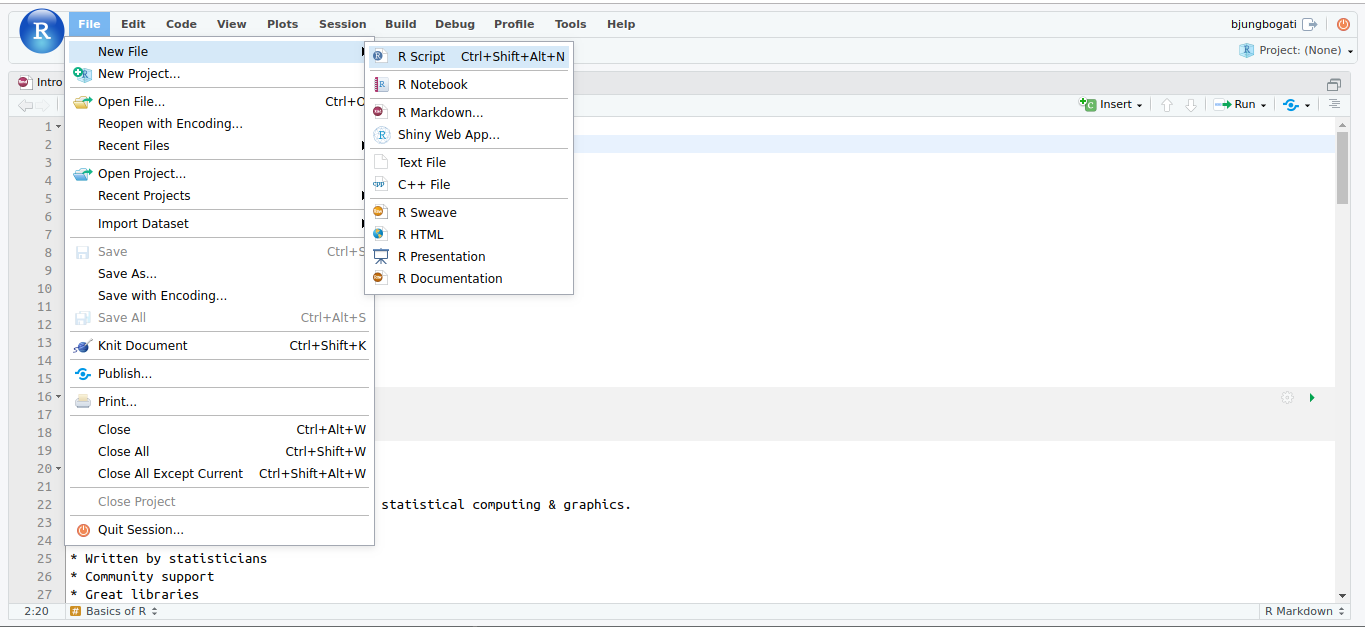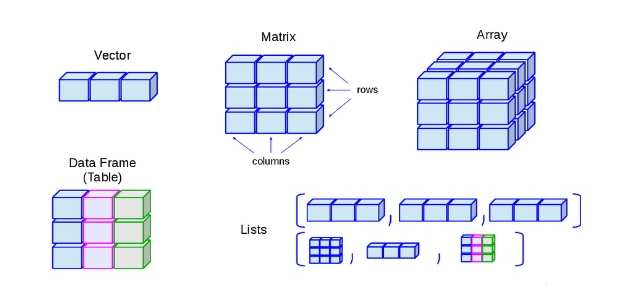# 1. Introduction to R

## a. What is R?

R is an open source programming language designed for statistical computing & graphics.

• Free to use
• Reproducible
• Community support
• Great libraries
• Diverse industries (health, academia to business sector)

Note: Whatever you’re trying to do, you’re probably not the first to try doing it R. Chances are good that someone has already written a package for that.

## b. Creating a New file

1. Go to File > R Script# 2. Basics of R

## a. R as Calculator

# ADD
5 + 10
##  15
# Subtract
15 - 5
##  10
# Multiply
5 * 10
##  50
# Divide
100 / 5
##  20

## b. Function

A function performs a specific task when called.

### Types of Function:

Types Functions
Built-in print(), sum(), mean()
User-defined eg: add.me()
# print your name
print("My name is Binod")  # what's yours?
##  "My name is Binod"
#add 1 to 100
sum(1:100)
##  5050
# mean of 5, 10, 15
mean(5, 10, 15)
##  5
# median
median(c(5, 10, 15)) 
##  10
# answer
sum(5, 10, 15)/3
##  10
# square root
sqrt(16)
##  4
# User-defined function - add.me()

add.me <- function (x, y) {
print(x + y)
}

add.me(1, 2)
##  3

## c. Assignment

• Process to store values
• The <- operator is used for assignment.
• We assign values in a variable.
total <- sum(1:50)
total
##  1275
avg <- mean(5, 10, 15)
avg
##  5

## d. Mutiplication Table

num = as.integer(readline(prompt = "Type a number: "))
## Type a number:
for(i in 1:10) {
print(paste(num,'x', i, '=', num * i))
}
##  "NA x 1 = NA"
##  "NA x 2 = NA"
##  "NA x 3 = NA"
##  "NA x 4 = NA"
##  "NA x 5 = NA"
##  "NA x 6 = NA"
##  "NA x 7 = NA"
##  "NA x 8 = NA"
##  "NA x 9 = NA"
##  "NA x 10 = NA"

# 3. Data Structures in R

To make best out of R, you need solid basics of data structures and how to operate on those.

To understand computations in R, two slogans are helpful:

• Everything that exists is an object.
• Everything that happens is a function call.
Dimension Type
- Homogeneous Heterogeneous
1D Vector List
2D Matrix Data Frame
nD Array* -

Note: Homogeneous allow only single data types.## 3.1 Vector

Vector is one-directional sequence of elements having same data types.

Type Example
Logical TRUE, FALSE
Integer 2L, 5L (L defines as integer)
Numeric 2, 5.5 (Double i.e ‘real number’)
Complex 1+3i
Character “apple”, “ball”

R provides many functions to examine features of vectors and other objects, for example

Function Description
class() what kind of object is it (high-level)?
typeof() what is the object’s data type (low-level)?
length() how long is it? What about two dimensional objects?
attributes() does it have any metadata?

### a. Creating a vector

color <- c("red", "orange", "blue")
color
##  "red"    "orange" "blue"

### b. Making different types of atomic vectors

student.name <- c("Anu", "Bikash", "Saroj")
student.age <- c(20, 24, 27)
student.gender <- c("Female", "Male", "Male")
student.married <- c(FALSE, FALSE, TRUE)
student.id <- 1:length(student.name)

Type checking:

typeof(student.age)
##  "double"
class(student.age)
##  "numeric"

## 3.2 List

A list is sequence of elements having same or different data types.

### a. Creating a list

lst <- list("Hello", 2, "All")

### b. Create a list

x <- 1:10
x <- as.list(x)

length(x) # check length of x
##  10
typeof(x) # check type of x
##  "list"

### c. Creating student’s list

student <- list(id = student.id,
name = student.name,
age = student.age,
gender = student.gender,
marital.status = student.married)

student
## $id ##  1 2 3 ## ##$name
##  "Anu"    "Bikash" "Saroj"
##
## $age ##  20 24 27 ## ##$gender
##  "Female" "Male"   "Male"
##
## $marital.status ##  FALSE FALSE TRUE ### d. Checking the list elements student # shows header and values ##$name
##  "Anu"    "Bikash" "Saroj"
student[] # shows age
##  20 24 27

## 3.3 Matrix

Matrix is a collection of data elements arranged in a two-dimensional rectangular layout.

### a. Creating a matrix

m <- matrix(1:9, nrow = 3)

# other ways to create matrix
m <- 1:10
dim(m) <- c(2, 5)

### b. Create two vectors x and y

x <- 1:10
y <- 11:20

### c. Combine two vectors by row or col

z_rowbind <- rbind(x, y)
z_colbind <- cbind(x, y)

### d. Check dimension of vectors

dim(z_rowbind)
##   2 10
dim(z_colbind)
##  10  2

## 3.4 Data Frame

Data frame is a list of vector having equal length and used for storing data tables.

### a. Creating data frame

df <- data.frame(id = 1:5,
name= c("Apple", "Ball",
"Cat", "Dog", "Elephant"))
df

### b. Creating student data frame

student.df <- data.frame(student, stringsAsFactors = F)

student.df

### c. Useful functions for Data Frame

Functions Description
head() see first 6 rows
tail() see last 6 rows
dim() see dimensions
nrow() number of rows
ncol() number of columns
str() structure of each column
names() will list the names attribute for a data frame (or any object really), which gives the column names.